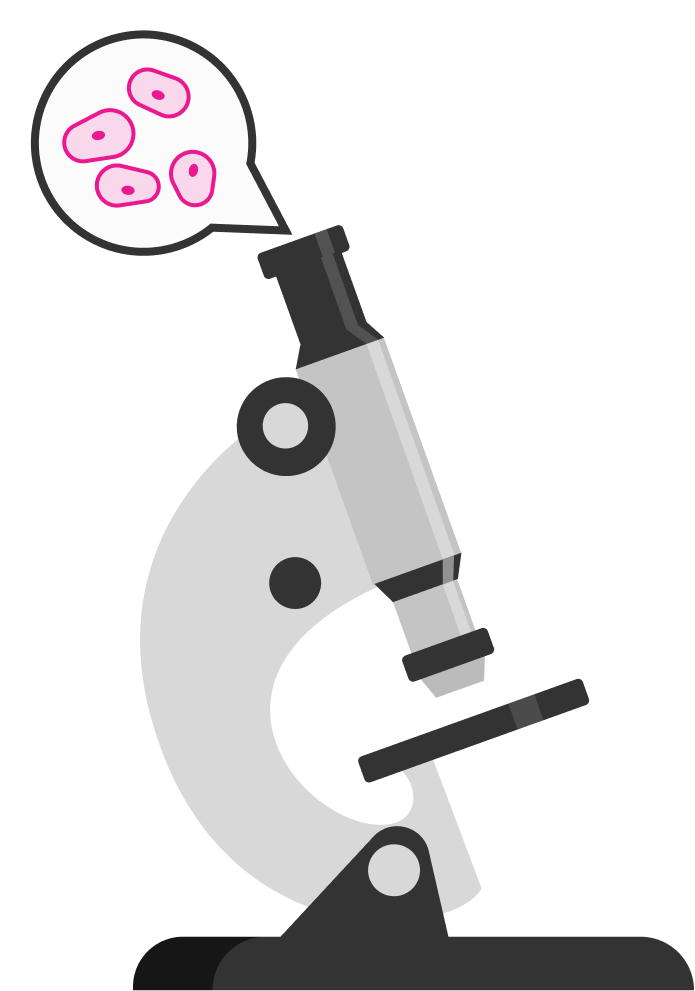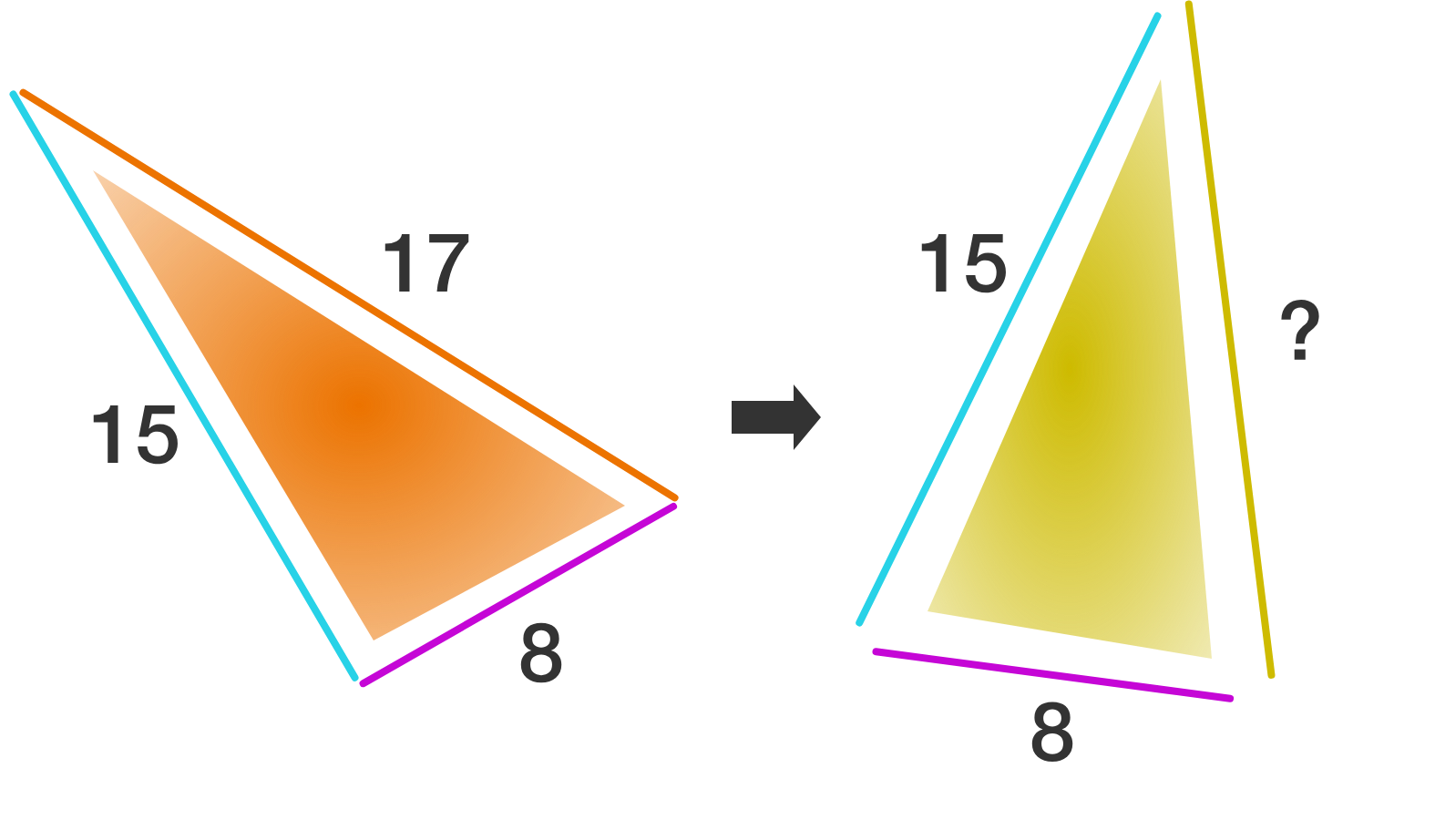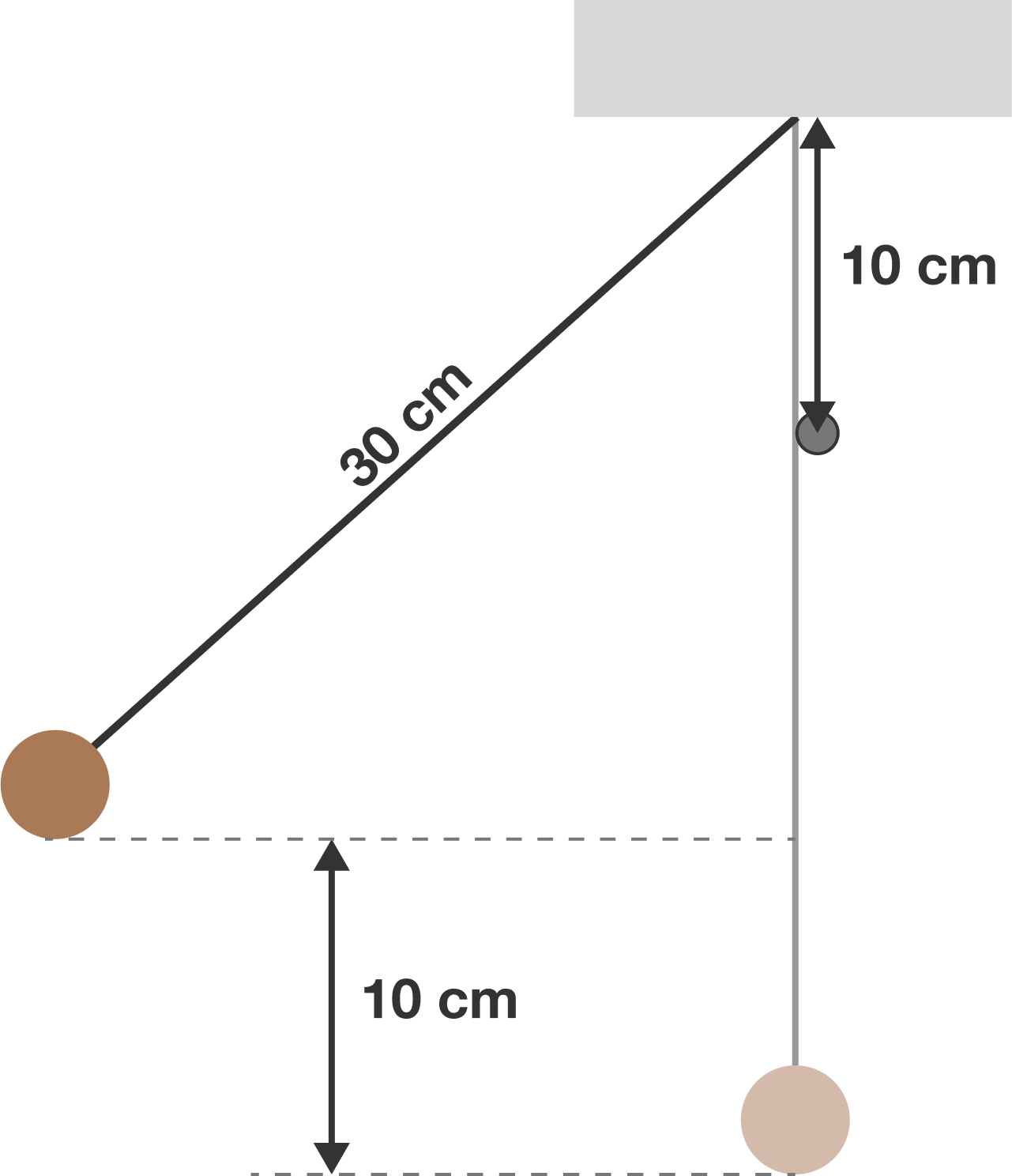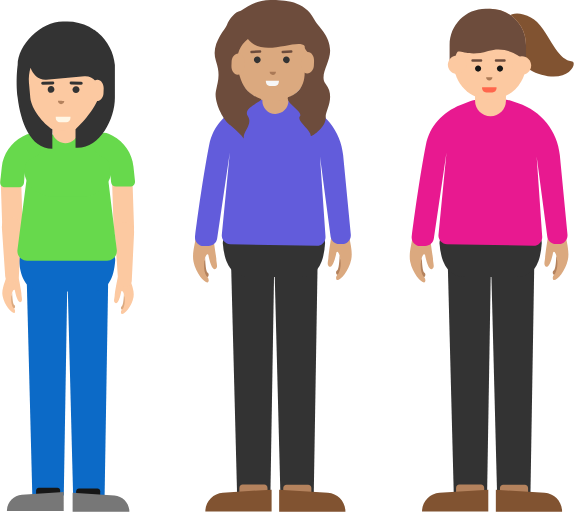# Problems of the Week

Contribute a problem

# 2018-10-15 BasicA biologist conducting experiments with animal cells wants to observe them under a microscope. Animal cells are semi-permeable, meaning that molecules like water and oxygen can move freely in and out of the cell, but charged ions cannot.

She prepares the slides by placing the cells in a 0.9% $\ce{NaCl}$ solution (to form a seal between the slide and the base).

What would happen if she used distilled water instead of the salt solution?

Two of the following statements are true and the other one is false:

1. This statement is true.
2. The first statement is false.
3. The second statement is true.

Which statement is false?

Bonus: Which statements would be false if only one of the statements is true?A triangle is made by connecting three sticks of lengths 8, 15, and 17.

Can you replace the stick of length 17 with one of a different length in order to make the area greater?A ball hangs from a $30 \text{ cm}$ string which is attached to the ceiling. A peg is nailed into the wall such that the string rests on the peg $10 \text{ cm}$ down from the ceiling. Then, the ball is held up to a height of $10 \text{ cm}$ from its lowest position (keeping the string taut), and then released.

Which of the following is true?

Note: Air resistance is negligible. Damping and other external forces should not be considered. Consider the ball and string to be a simple pendulum.

Ella, Bella, and Frabduzella are all different heights.

If Ella is taller than Bella, what is the probability that Ella is taller than Frabduzella?Assume that for any particular height, each girl has the same chance to be that height.

Hint: This conditional probability wiki may prove helpful!

×